# Machine Learning Training in Mumbai

## Machine Learning Training In Mumbai | TryCatch Classes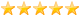4.9 out of 5 based on 249 ratings.
300 user reviews.

Are you looking for the Best Machine Learning Institute In Mumbai? TryCatch Classes is the best ML classes in Borivali, Mumbai. Learn to create Machine Learning Algorithms in Python from Data Science experts with private one-on-one classroom machine learning training in Mumbai.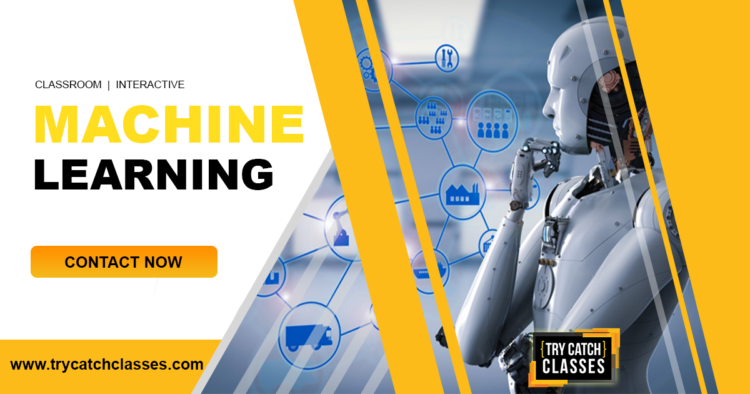#### WHAT YOU WILL LEARN:

• Master Machine Learning on Python.
• Make accurate predictions.
• Make powerful analysis.
• Learn to create robust Machine Learning models.
• Handle specific topics like Reinforcement Learning, NLP and Deep Learning.
• Handle advanced techniques like Dimensionality Reduction.
• Know which Machine Learning model to choose for each type of problem.
• Build an army of powerful Machine Learning models and know how to combine them to solve any problem.

#### PREREQUISITES:

In order to learn Machine Learning, here is the list of prerequisites you need to know:

• Basic Knowledge of Python is helpful.
• Some prior coding or scripting experience is required.
• At least high school level math will be required.

#### COURSE MODULE:

Here is the course module for Machine Learning:

#### Machine Learning Development Course Content

Module 1:
Introduction to Machine Learning (ML)
• What is Machine Learning ?
• Use Cases of Machine Learning.
• Types of Machine Learning – Supervised to Unsupervised methods.
• Machine Learning workflow.
Module 2:
Statistics for Data Science
• Common charts used.
• Inferential Statistics.
• Probability, Central Limit theorem, Normal Distribution & Hypothesis testing.
Module 3:
Data Visualization
• Plotting basic statistical charts in Python.
• Data visualization with Matplotlib.
• Statistical data visualization with Seaborn.
• Interactive data visualization with Bokeh.
Module 4:
Exploratory Data Analysis
• Introduction to Exploratory Data Analysis (EDA) steps.
• Plots to explore relationship between two variables.
• Histograms, Box plots to explore a single variable.
• Heat maps, Pair plots to explore correlations.
Module 5:
Data Preprocessing
• Preprocessing techniques like missing value imputation.
• Encoding categorical variables.
• Scaling, Too many nulls, Same values/skew.
• Data types, Missing value imputation.
• When column doesn’t have missing values.
• Categorical Attributes, Related Attributes.
Module 6:
Linear Regression
• Introduction to Linear Regression.
• Use cases of Linear Regression.
• How to fit a Linear Regression model?
• Evaluating and interpreting results from Linear Regression models.
• Project:
• How linear regression helps determine demand in Restaurant.
Module 7:
Logistic Regression
• Introduction to Logistic Regression.
• Logistic Regression use cases.
• Understand use of odds & Logit function to perform logistic regression.
• Project:
• Predicting default cases in the Banking Industry.
Module 8:
Decision trees & Random Forests
• Introduction to Decision Trees & Random Forest .
• Understanding criterion(Entropy & Information Gain) used in Decision Trees.
• Using Ensemble methods in Decision Trees.
• Applications of Random Forest.
• Project:
• Predict passengers survival in a Ship mishap.
Module 9:
Model evaluation techniques
• Introduction to evaluation metrics and model selection in Machine Learning.
• Importance of Confusion matrix for predictions.
• Measures of model evaluation – Sensitivity, specificity, precision, recall & f-score.
• Use AUC-ROC curve to decide best model.
• K-fold Cross Validation.
• Parameter Tuning.
• Grid Search.
• XGBoost.
• Project:
• Applying model evaluation techniques to prior projects.
Module 10:
Dimensionality Reduction using PCA
• Introduction to KNN.
• Calculate neighbours using distance measures.
• Find optimal value of K in KNN method.
• Project:
• Optimize model performance using PCA on high dimension dataset.
Module 11:
KNN (K – Nearest neighbours)
• Unsupervised Learning: Introduction to Curse of Dimensionality.
• What is dimensionality reduction?
• Technique used in PCA to reduce dimensions.
• Applications of Principle component Analysis (PCA).
• Project:
• Classify smokers among
• Classify malicious websites using close neighbour technique.
• Credit scoring analysis using weighted k nearest neighbor
Module 12:
Naïve Bayes classifier
• Introduction to Naïve Bayes classification.
• Refresher on Probability theory.
• Applications of Naive Bayes Algorithm in Machine Learning.
• Project:
• Classify Spam SMS, based on probability.
Module 13:
K-means clustering technique
• Introduction to K-means clustering.
• Decide clusters by adjusting centroids.
• Find optimal ‘k value’ in kmeans.
• Understand applications of clustering in Machine Learning.
• Project:
• Predict flower species in Iris flower data.
Module 14:
Support vectormachines (SVM)
• Introduction to SVM.
• Figure decision boundaries using support vectors.
• Find optimal ‘k value’ in kmeans.
• Applications of SVM in Machine Learning.
• Project:
• Predicting wine quality without tasting the wine.
• Personal Info App.
Module 15:
Time series forecasting
• Introduction to Time Series analysis.
• Stationary vs non stationary data.
• Components of time series data.
• Interpreting autocorrelation & partial autocorrelation functions.
• Stationarize data and implement ARIMA model.
• Project:
• Forecast demand for Air travel.
Module 16:
Ensemble learning
• Introduction to Ensemble Learning.
• What are Bagging and Boosting techniques?
• What is Bias variance trade off?
• Project:
• Predict annual income classes from adult census data.
• Personal Info App.
Module 17 :
Stacking
• Introduction to stacking.
• Use Cases of stacking.
• How stacking improves machine learning models?
• Project:
• Predict survivors in Titanic case
Module 18 :
Optimization
• Introduction to optimization in ML.
• Applications of optimization methods.
• Optimization techniques: Linear Programming using Excel solver.
• How Stochastic Gradient Descent(SGD) Works?
• Project:
• Apply SGD on Regression data (sklearn dataset).
• Personal Info App.
#####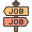PLACEMENTS:

We offer unique placement assistance in Machine Learning Training and that is why we are the no 1 Machine Learning training classes in Mumbai. At TryCatch Classes, we provide interview questions and prepare you for the interview as well. So you’ll get the best placement assistance for Machine Learning.

#####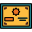CERTIFICATION:

At the end of the Machine Learning course in Mumbai, you will get a Certification from us which will be very helpful for you in your future. Our certification is valid everywhere on the globe because we are the best machine learning training institute in Mumbai.

#####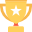AWARDS:

TryCatch Classes has been awarded multiple times as the “Most Promising Software Training Institute in Maharashtra” and “Social Impact Award for Outstanding Contribution to Indian Education System” at Education several Award functions.

#### OTHER RELATED COURSES:

TryCatch Classes provides the best Machine Learning(Python) Training in Mumbai. Along with Machine Learning(Python) Training Course, you can also learn,